# Cumulative Sum in MATLAB

• Last Updated : 18 Oct, 2021

The cumulative sum of a sequence is a running sums or partial sums of a sequence.

The cumulative sums of the sequence {a,b,c,…}, are a, a+b, a+b+c, ….

MATLAB allows us to calculate the cumulative sum of a vector, matrix using cumsum() method. Different syntax of cumsum() method are:

• B = cumsum(A)
• B = cumsum(A,dim)
• B = cumsum(___,direction)
• B = cumsum(___,nanflag)

Let us discuss the above syntax in detail:

### cumsum(A)

• The method returns the cumulative sum of A starting at the beginning of array A.
• If A is a vector, then it returns the cumulative sum of sequence A
• If A is a matrix, then it returns the cumulative sum along each column of A.

Example 1:

## Matlab

 `% Input vector``A = 2:8;` `B = cumsum(A);` `% Displays cumulative sums``% of A``disp(B)`

Output :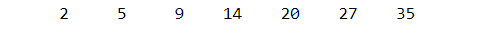Example 2:

## Matlab

 `% Input matrix``A = [1 4 7; 2 5 8; 3 6 9];``disp(``"Matrix :"``)``disp(A)` `B = cumsum(A);` `% Display cumulative sum of matrix A``disp(``"Cumulative sum :"``)``disp(B)`

Output :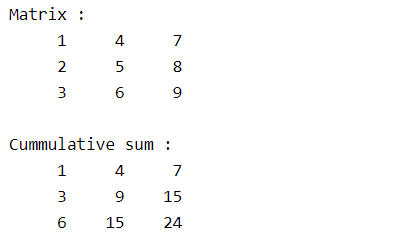### cumsum(A,dim)

• Returns the cumulative sum of matrix A along with each dim.
• dim takes two values 1 or 2.
• dim = 1, refers to along each column.
• dim = 2, refers along each row.

## Matlab

 `% input matrix``A = [1 3 5; 2 4 6];``disp(``"Matrix :"``)``disp(A)` `% Cumulative sum along each``% row from left to right``B = cumsum(A,2);``disp(``"Cumulative sum :"``)``disp(B)`

Output :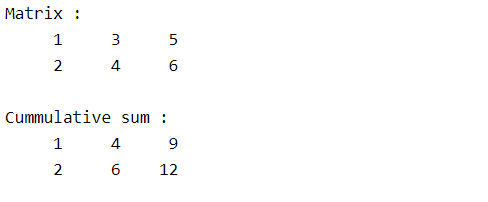### cumsum(___,direction)

• Returns the cumulative sum of the input vector or matrix in the given direction.
• direction takes two values ‘forward’ or ‘reverse’
• If the direction is ‘reverse’, then calculates cumulative sum in reverse i.e if we are considering matrix along each column, then it returns cumulative sum starting from bottom to top of each column.

## Matlab

 `% input matrix``A = [1 3 5; 2 4 6];``disp(``"Matrix :"``)``disp(A)` `% Cumulative sum of A along each``% row starting from right to left``B = cumsum(A,2,``'reverse'``);``disp(``"Cumulative sum :"``)``disp(B)`

Output :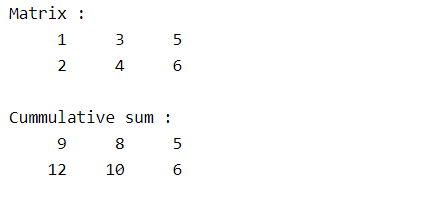### cumsum(___,nanflag)

• nanflag value decides whether to include or exclude NaN value of the vector in cumulative sum or not.
• nanflag takes two values ‘includenan’ or ‘omitnan’ corresponds to including NaN elements and excluding NaN elements respectively.
• ‘omitNaN’ considers NaN values as 0.

Note : NaN + number = NaN

## Matlab

 `% Input vector``A = [3 5 NaN 9 0 NaN];``disp(``"Vector :"``);``disp(A);` `% Including NaN values``B = cumsum(A,``'includenan'``);``disp(``"Cumulative sum Include NaN :"``);``disp(B);` `% Excluding NaN values``B = cumsum(A,``'omitnan'``);``disp(``"Cumulative sum Exclude NaN :"``);``disp(B);`

Output :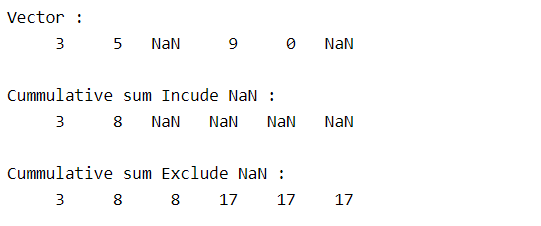My Personal Notes arrow_drop_up